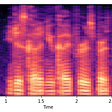# Introduction

Fuzzy logic principles can be used to cluster multidimensional data, assigning each point a membership in each cluster center from 0 to 100 percent. This can be very powerful compared to traditional hard-threshold clustering where every point is assigned a crisp, exact label. This algorithm works by assigning membership to each data point corresponding to each cluster center on the basis of distance between the cluster center and the data point. More the data is near to the cluster center more is its membership towards the particular cluster center. Clearly, summation of membership of each data point should be equal to one.

It is an unsupervised clustering algorithm that permits us to build a fuzzy partition from data. The algorithm depends on a parameter m which corresponds to the degree of fuzziness of the solution. Large values of m will blur the classes and all elements tend to belong to all clusters. The solutions of the optimization problem depend on the parameter m. That is, different selections of m will typically lead to different partitions. Given below is a gif that shows the effect of the selection of m obtained from the fuzzy c-means.

# K-Means versus Fuzzy C-Means

Let us compare these two powerful algorithms to get a clear idea of where the fuzzy c-means algorithm fits in.

1. Attribution to a cluster: In fuzzy clustering, each point has a probability of belonging to each cluster, rather than completely belonging to just one cluster as it is the case in the traditional k-means. In Fuzzy-C Means clustering, each point has a weighting associated with a particular cluster, so a point doesn’t sit “in a cluster” as much as has a weak or strong association to the cluster, which is determined by the inverse distance to the center of the cluster.
2. Speed: Fuzzy-C means will tend to run slower than K means, since it’s actually doing more work. Each point is evaluated with each cluster, and more operations are involved in each evaluation. K-Means just needs to do a distance calculation, whereas fuzzy c means needs to do a full inverse-distance weighting.
3. Personal Opinion: FCM/Soft-K-Means is “less stupid” than Hard-K-Means when it comes to elongated clusters (when points otherwise consistent in other dimensions tend to scatter along a particular dimension or two).

We should realize that fuzzy c-means is a special case of K-means when the probability function used is simply 1 if the data point is closest to a centroid and 0 otherwise.

# Steps in Fuzzy C-Means

The process flow of fuzzy c-means is enumerated below:

1. Assume a fixed number of clusters k.
2. Initialization: Randomly initialize the k-means μk associated with the clusters and compute the probability that each data point xi is a member of a given cluster k, P(point xi has label k|xi, k).
3. Iteration: Recalculate the centroid of the cluster as the weighted centroid given the probabilities of membership of all data points xi:

4. Termination: Iterate until convergence or until a user-specified number of iterations has been reached (the iteration may be trapped at some local maxima or minima).

Implementation in python can be found here. A mathematical explanation can be found here.

# Evaluation Metrics for Clusters

Most of the measures used to evaluate the clusters under other clustering algorithms can be used for Fuzzy C-Means. Even though these methods rely on the subject matter of the expert, I am listing below some popular measures used to evaluate the clusters so formed:

1. Homogeneity analysis of the clusters formed.
2. The clusters thus formed using Fuzzy C-Means, need to homogeneous and separated from other clusters.
3. Coefficient of Variance analysis for each cluster.
4. Pearson Correlation can be used for validating the quality of clusters.
5. If we have ground truth cluster values, precision, recall, and f-score can also be considered.
6. Elbow Method and Silhouette are also some statistical measures for evaluating your clusters (I would rather use them to in pre-definition of cluster number).
7. Entropy-based methods — Research Paper

# Pros and Cons

Now comes the time to evaluate the algorithm itself!

## Pros

1. Gives best result for overlapped data set and comparatively better then k-means algorithm.
2. Unlike k-means where data point must exclusively belong to one cluster center here data point is assigned membership to each cluster center as a result of which data point may belong to more then one cluster center.

## Cons

1. Apriori specification of the number of clusters.
2. With lower value of β we get the better result but at the expense of more number of iteration.
3. Euclidean distance measures can unequally weight underlying factors.
4. The performance of the FCM algorithm depends on the selection of the initial cluster center and/or the initial membership value.

# Improvements

Many improvements have been made to fuzzy c-means like Self-Adaptive Fuzzy c-Means Algorithm for Determining the Optimal Number of Clusters, Random projections fuzzy c-means (RPFCM) for big data clustering, Extended Fuzzy C-Means with Random Sampling Techniques for Clustering Large Data, Fuzzy C-means++: Fuzzy C-means with effective seeding initialization, etc., which make this algorithm more powerful.

I hope this article helps you to get an insight into fuzzy c-means algorithm. I am looking forward to your suggestions.

--

--

--

## More from Geek Culture

A new tech publication by Start it up (https://medium.com/swlh).

## Comparing Facebook’s M2M to mT5 in low resources translation (English-Yoruba).## Beyond Neural Networks## K-means Clustering & its real use-cases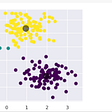## Linear Regression: Everything From Math to Program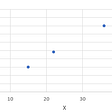## Feature Scaling and Normalisation in a Nutshell## A Hebbian cell assembly is formed at full strength on a single trial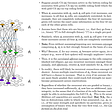## A Deep Learning Approach to Enhance 3D City Models## Aman Gupta

Is a pantomath and a former entrepreneur. Currently, he is in a harmonious and a symbiotic relationship with Data.

## Music Genre Classification## Predicting solar power output using machine learning techniques## Evaluating classification models with Accuracy, Precision and Recall.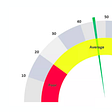## Age prediction of a speaker’s voice